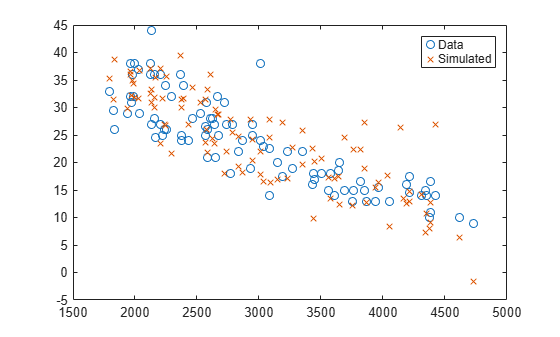Documentation

### This is machine translation

Mouseover text to see original. Click the button below to return to the English version of the page.

Note: This page has been translated by MathWorks. Click here to see
To view all translated materials including this page, select Country from the country navigator on the bottom of this page.

# random

Simulate responses with random noise for linear regression model

## Syntax

``ysim = random(mdl,Xnew)``

## Description

example

````ysim = random(mdl,Xnew)` simulates responses to the predictor data in `Xnew` using the linear model `mdl`, adding random noise.```

## Examples

collapse all

Create a quadratic model of car mileage as a function of weight from the `carsmall` data set.

```load carsmall X = Weight; y = MPG; mdl = fitlm(X,y,'quadratic');```

Create simulated responses to the data with random noise.

`ysim = random(mdl,X);`

Plot the original responses and the simulated responses to see how they differ.

```plot(X,y,'o',X,ysim,'x') legend('Data','Simulated')```## Input Arguments

collapse all

Linear regression model object, specified as a `LinearModel` object created by using `fitlm` or `stepwiselm`, or a `CompactLinearModel` object created by using `compact`.

New predictor input values, specified as a table, dataset array, or matrix. Each row of `Xnew` corresponds to one observation, and each column corresponds to one variable.

• If `Xnew` is a table or dataset array, it must contain predictors that have the same predictor names as in the `PredictorNames` property of `mdl`.

• If `Xnew` is a matrix, it must have the same number of variables (columns) in the same order as the predictor input used to create `mdl`. Note that `Xnew` must also contain any predictor variables that are not used as predictors in the fitted model. Also, all variables used in creating `mdl` must be numeric or logical. To treat numerical predictors as categorical, identify the predictors using the `'CategoricalVars'` name-value pair argument when you create `mdl`.

Data Types: `single` | `double` | `table`

## Output Arguments

collapse all

Simulated response value, returned as a numeric vector. The simulated value is the predicted response values at `Xnew` perturbed by random noise. The noise is independent and normally distributed, with mean equal to zero and variance equal to the estimated error variance of the model.

## Alternative Functionality

For predictions without random noise, use `predict` or `feval`. These two functions give the same predictions.

• `predict` uses a single input argument with one observation in each row, and gives confidence intervals on its predictions.

• `feval` uses multiple input arguments with one input for each predictor variable.